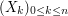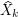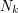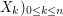## A quantization algorithm for solving multidimensional optimal stopping problems, preprint

 Title A quantization algorithm for solving multidimensional optimal stopping problems, preprint Publication Type Conference Paper Year of Publication 2001 Authors Vlad Bally, and Gilles Pagès Conference Name University de Paris VI Abstract A new grid method for computing the Snell envelope of a function of an-valued simulatable Markov chainis proposed. (This is a typical nonlinear problem that cannot be solved by the standard Monte Carlo method.) Everyis replaced by a 'quantized approximation'taking its values in a gridof size. Thegrids and their transition probability matrices form a discrete tree on which a pseudo-Snell envelope is devised by mimicking the regular dynamic programming formula. Using the quantization theory of random vectors, we show the existence of a set of optimal grids, given the total numberof elementary-valued quantizers. A recursive stochastic gradient algorithm, based on simulations of (, yields these optimal grids and their transition probability matrices. Some a priori error estimates based on the-quantization errorsare established. These results are applied to the computation of the Snell envelope of a diffusion approximated by its (Gaussian) Euler scheme. We apply these results to provide a discretization scheme for reflected backward stochastic differential equations. Finally, a numerical experiment is carried out on a two-dimensional American option pricing problem.